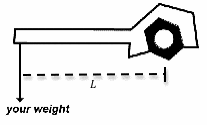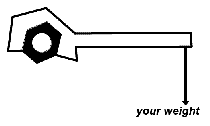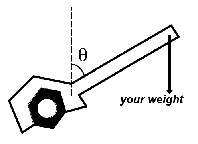# Torque on a wrench

## Homework Statement

(a) Suppose you have just barely loosened a rusty bolt. Your mass is 80 kg and you have a wrench of length L = 50 cm placed as shown in the diagram below, and hung off the end so all your weight was applied, in the downward direction, to the end of the wrench. What is the magnitude and direction of the torque?
magnitude 392 N m
direction counterclockwise(b) Now suppose you had a bolt that needed to be tightened to 250 N · m. You place the wrench as shown in the diagram below, and hung off the end so all your weight was applied, in the downward direction, to the end of the wrench. What length wrench would you need to tighten the bolt completely?
L = .3189 m(c) Suppose you had the same wrench as in part (a), but you placed the wrench at an angle θ = 54° with the vertical, as shown below. How much torque is applied to the bolt?
magnitude
direction clockwise## Homework Equations

Torque=radius x force (in this case, weight) x sin(theta)

## The Attempt at a Solution

I am having difficulty with the 3rd part. If the torque is as the above equation,
T=.5 m x 80kg x 9.8 m/s2 x sin(54)
=-219.0
which magnitude is 219 N m with a clockwise direction
However, this is incorrect. Perhaps this is my angle-do I need to subtract 54 from 90? How do you know what angle to look at? Where do you form the right triangle for the correct trig?

haruspex
Homework Helper
Gold Member
2020 Award
T=.5 m x 80kg x 9.8 m/s2 x sin(54)
=-219.0
The algebra is right, but you should have noticed it ought to give a positive answer. (To get the expected negative sign for the torque you would need to put in the appropriate sign on the angle, i.e. -54 degrees.)
What did you forget to do when you entered the angle into the sine function on your calculator?

The algebra is right, but you should have noticed it ought to give a positive answer. (To get the expected negative sign for the torque you would need to put in the appropriate sign on the angle, i.e. -54 degrees.)
What did you forget to do when you entered the angle into the sine function on your calculator?
hmm...my calculator was in radian mode. I need degree mode, don't I? ha

haruspex
Homework Helper
Gold Member
2020 Award
hmm...my calculator was in radian mode. I need degree mode, don't I? ha
Right.

With regard to deciding when to take sine and when cosine, I always check by considering a corner case, i.e. the angle being 0 or 90. Would the value of the trig function make sense in context? In the present case, when the angle is a right angle you would get maximum torque, so sine is right.

Right.

With regard to deciding when to take sine and when cosine, I always check by considering a corner case, i.e. the angle being 0 or 90. Would the value of the trig function make sense in context? In the present case, when the angle is a right angle you would get maximum torque, so sine is right.

Thank you! That was most helpful.